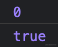# 前言

`Performance`版的`React`

# Preact 簡介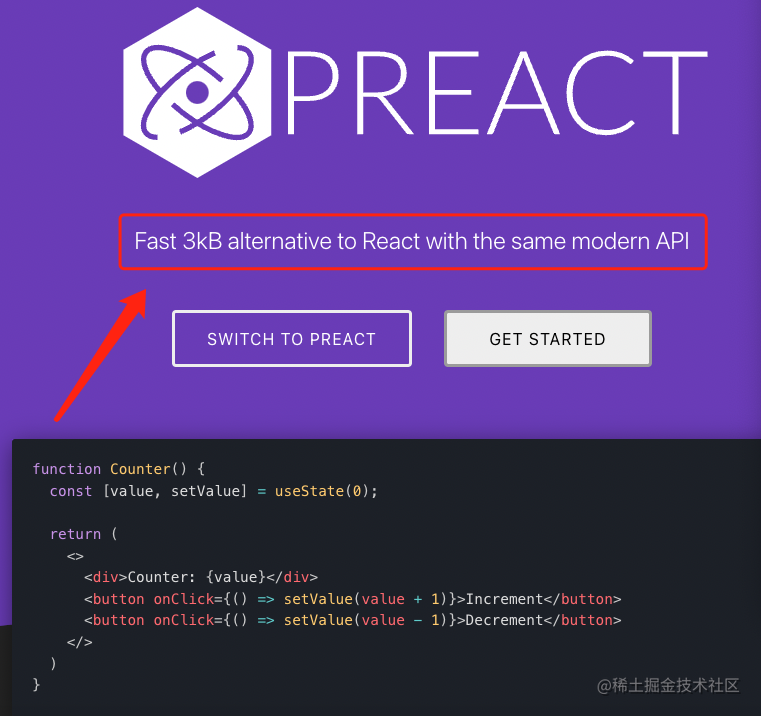```js npm create vite```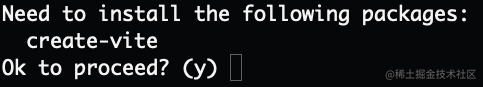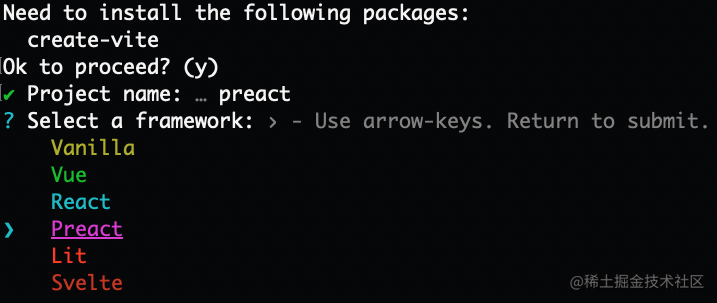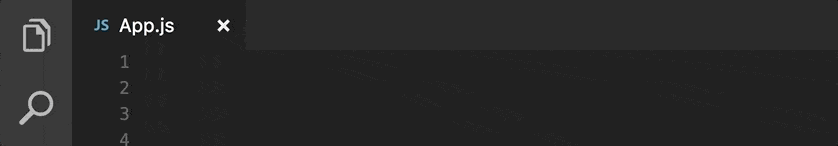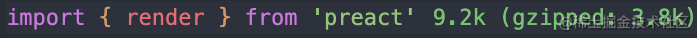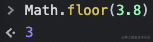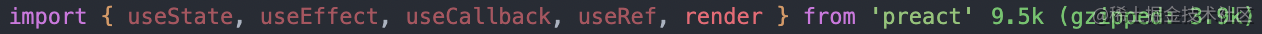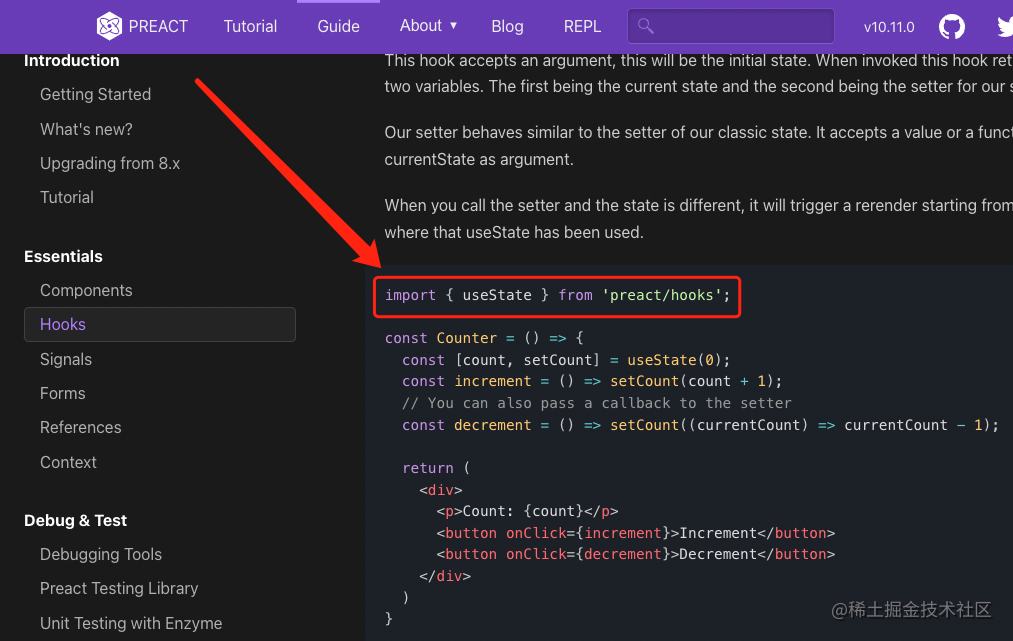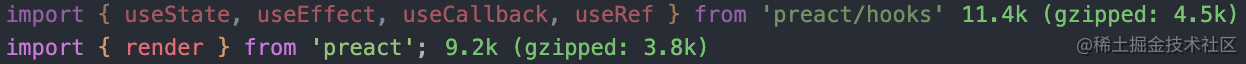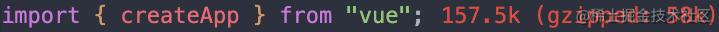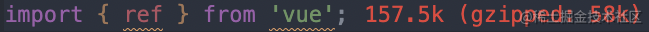# Preact Signals

```js import { signal, computed } from "@preact/signals";

const count = signal(0); const double = computed(() => count.value * 2);

function Counter() { return ( ); } ```

```html

```

`hooks`不同，`signals`可以在元件內部或外部使用。`signals`在類元件也可以很好的執行，因此您可以按照自己的節奏引入它們，並根據現狀，在幾個元件中試用它們，並隨著時間的推移逐漸採用它們。—— `Preact團隊`• 易衝突的全域性狀態
• 混亂的上下文
• 尋求更好的狀態管理
• 卓越的效能• 避免了`React Hooks`的一些心智負擔
• 效能比`React`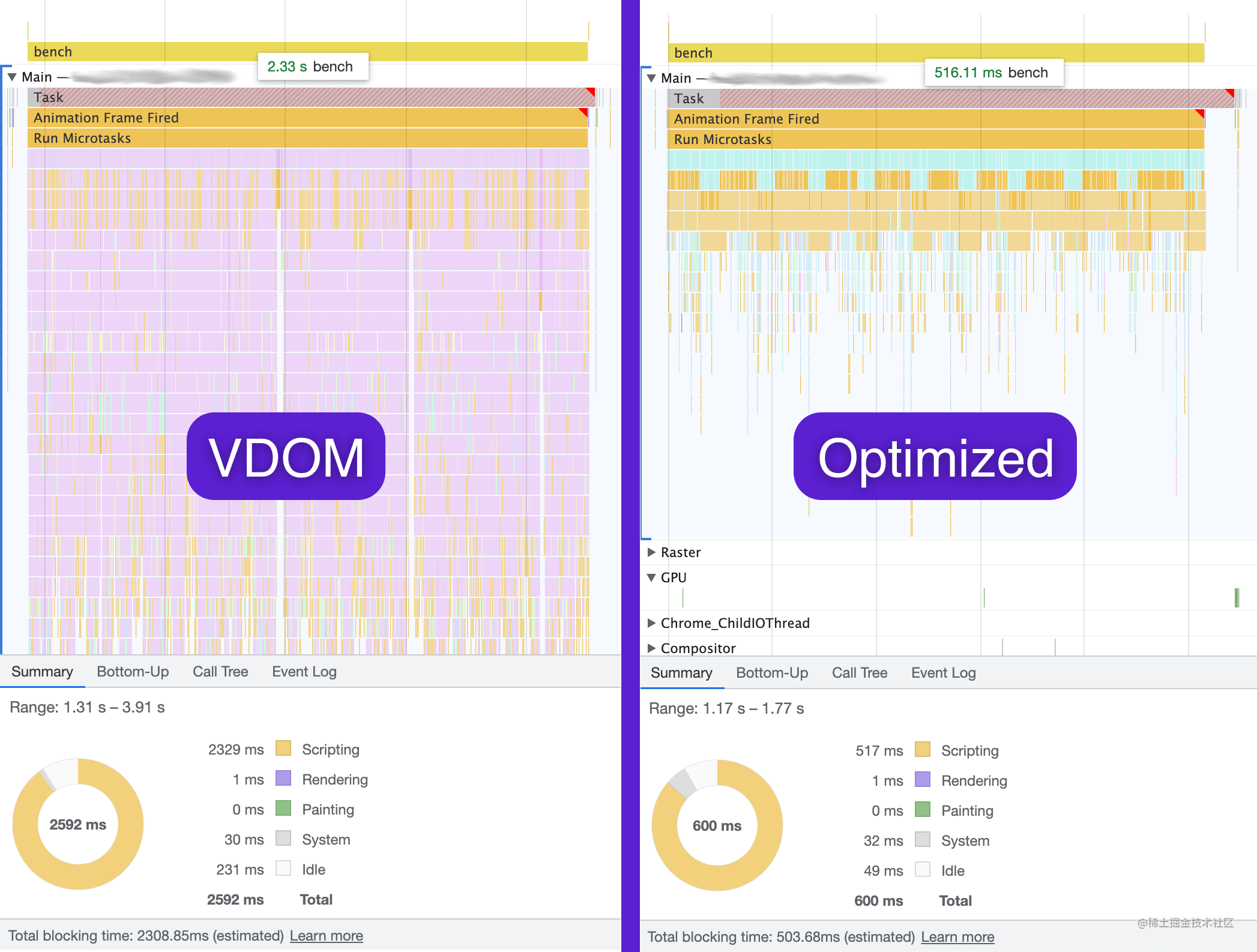`Preact`的老師`React`有在`React`裡實現`Vue`的計劃嗎？答案是否定的，自從`Preact Signals`釋出後大家就瘋狂`@Dan``Dan`看完後直接來了句：這與`React`的發展理念不是很吻合。(潛臺詞：我們才不會在`React`裡實現`Vue`呢)

`Angular`用裝飾器用的好好的那是因為人家強制要求使用`TS`，但`Vue`顯然不可能這樣做。而且為了防止未來裝飾器有變動（其實最近已經`Stage3`的裝飾器已經和`TS`裝飾器不一樣了），許多曾經使用裝飾器語法的庫為了規避這個風險也已經改用了別的寫法，如：`MobX``React DnD`等…# 用法

`Preact`版的`composition api`主要分為三個部分：

• `@preact/signals-core`
• `@preact/signals`
• `@preact/signals-react`

```js import { signal } from "@preact/signals-core";

const counter = signal(0);

// Read value from signal, logs: 0 console.log(counter.value);

// Write to a signal counter.value = 1; ```

```js const counter = signal(0); const effectCount = signal(0);

effect(() => { console.log(counter.value);

// Whenever this effect is triggered, increase `effectCount`. // But we don't want this signal to react to `effectCount` effectCount.value = effectCount.peek() + 1; }); ```

`composition api``@vue/composition-api`又有啥區別呢？區別就是`composition api`只是一個概念，而`@vue/composition-api`是一個實現了`composition api`的專案。當初尤雨溪提出`composition api`的時候(那時候還不叫`composition api`，好像叫什麼`functional base api`)遭到了大量質疑的聲音，於是有個大佬就用`Vue2`現有的`API`實現了一版尤雨溪的提案，尤雨溪覺得這玩意非常不錯！你們老噴我是因為你們沒有體驗過函式式的好，你們先用用試試，試完了保證你們直呼真香！於是聯絡該作者把`Vue2`版的`composition api`合併到`Vue`的倉庫中併發布為`@vue/composition-api`。但誰也不會用愛發電對不，剛開始當個娛樂專案給你宣傳了，時間一長也沒啥收益，該作者也就不維護了。此時另一位大佬出現了，他說既然沒人維護了那就交給我吧！他就是肝帝`AntFu`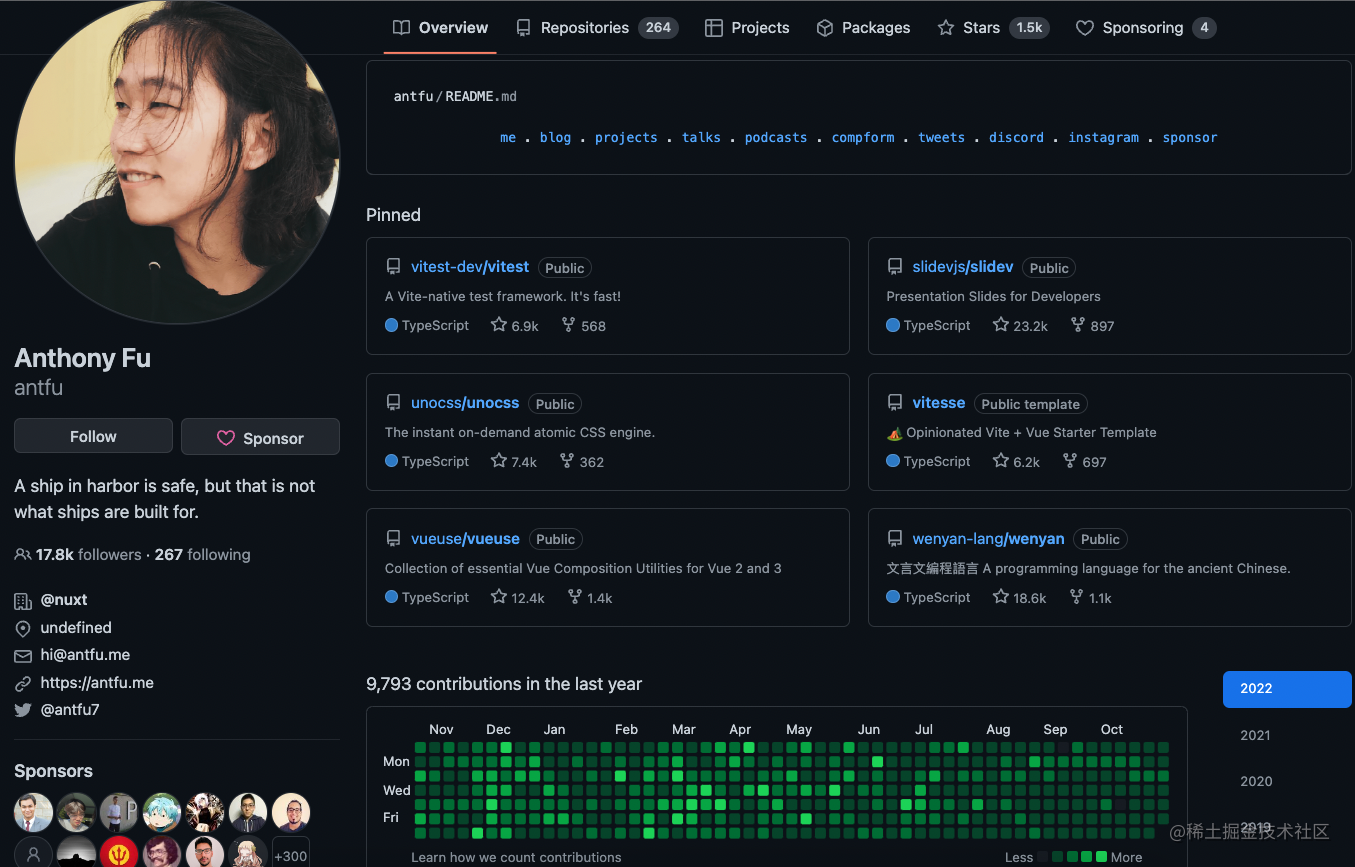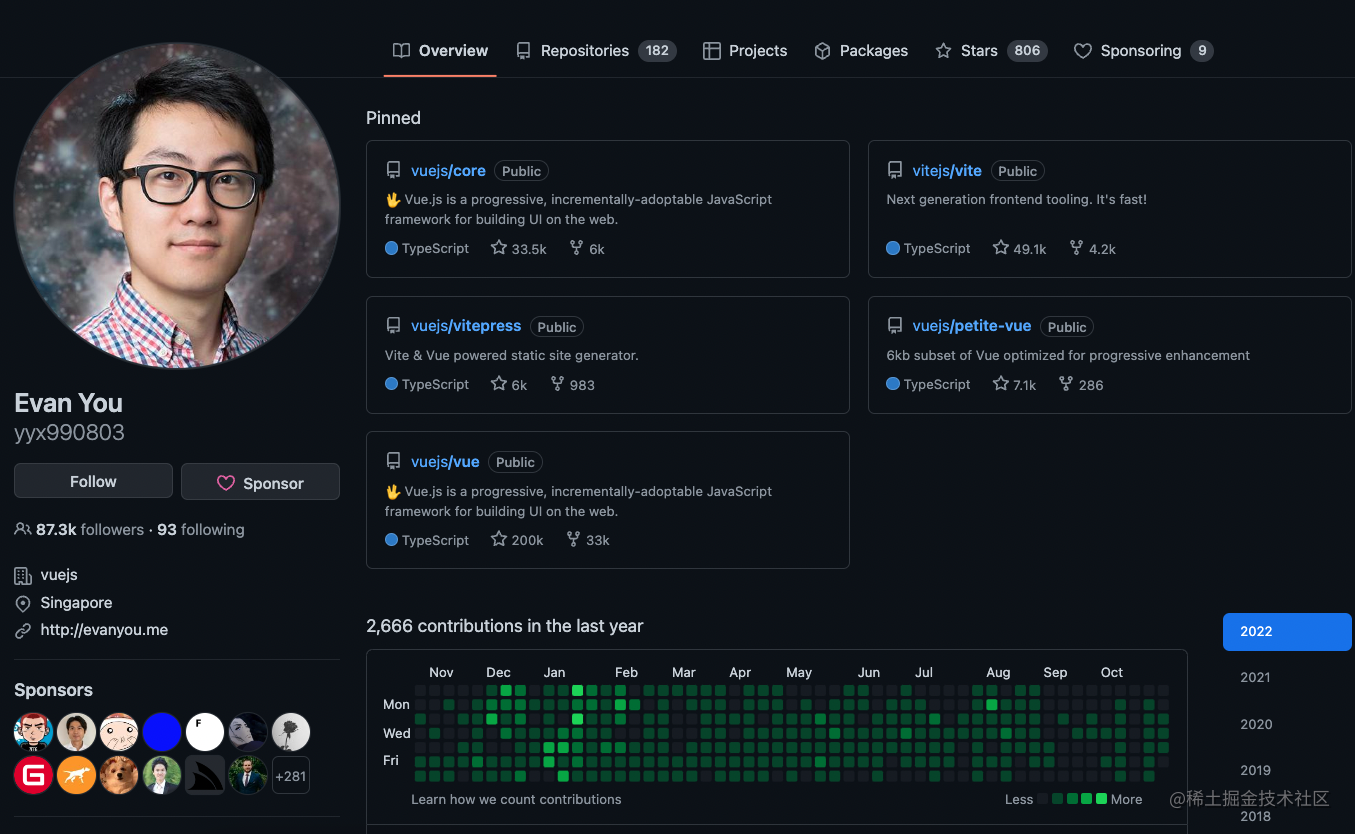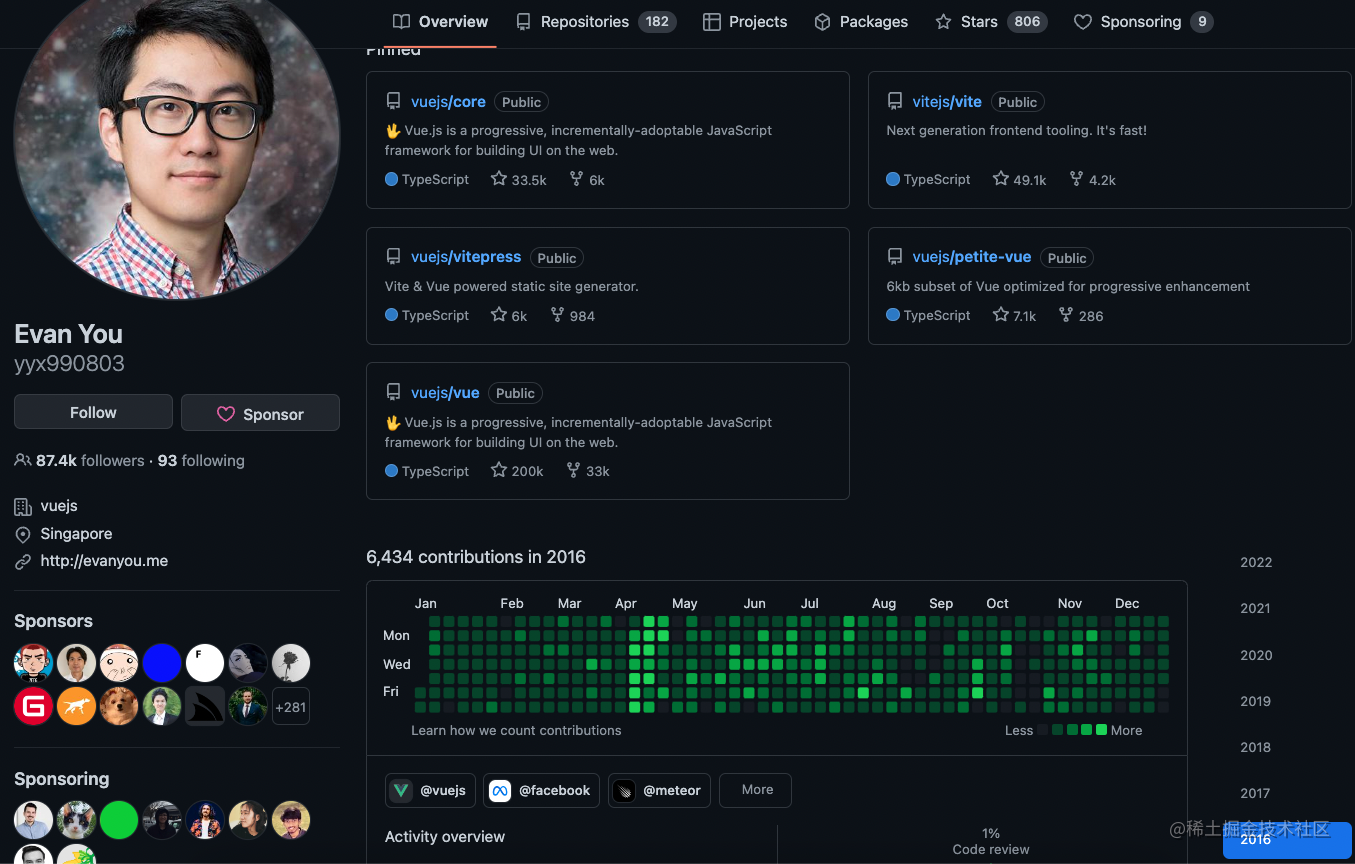```js import { ref, watchEffect } from 'vue';

const a = ref(0); const b = ref(0);

watchEffect(() => { console.log(a.value);

b.value++; }); ```

```js import { ref, watch } from 'vue';

const a = ref(0); const b = ref(0);

watch(a, value => { console.log(value);

b.value++; }); ```

```js import { ref, watch } from 'vue';

const a = ref(0); const b = ref(0);

watch([a, b], ([valueA, valueB]) => { console.log(valueA);

b.value = valueB + 1; }); ```

`Vue`的方案是既提供一個自動收集依賴的`watchEffect`，同時也提供一個手動收集依賴的`watch`

`Preact`的方案則是隻提供一個`effect`(類似`Vue``watchEffect`)，如果你寫出類似上面那樣的程式碼：

```js import { signal, effect } from '@preact/signals-core';

const a = signal(0); const b = signal(0);

effect(() => { console.log(a.value);

b.value++; }); ```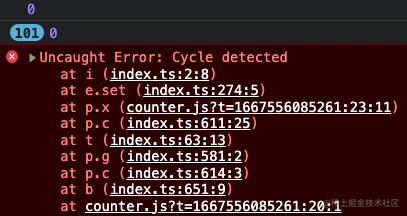《尤雨溪國外教程：親手帶你寫個簡易版的Vue！》

`Preact`沒做這樣的處理怎麼辦呢？那我們就避免在`effect`裡既對`signal`進行取值操作同時又對它進行賦值操作唄！```js import { createSignal, createEffect, untrack } from "solid-js";

const [a, setA] = createSignal(0); const [b, setB] = createSignal(0);

createEffect(() => { console.log(a()); console.log(untrack(b)); }); ```

```js import { signal, effect } from '@preact/signals-core';

const a = signal(0); const b = signal(0);

effect(() => { console.log(a.value);

b.value = b.peek() + 1; }); ``````js import { signal, computed } from "@preact/signals-core";

const name = signal("Jane"); const surname = signal("Doe");

const fullName = computed(() => name.value + " " + surname.value);

// Logs: "Jane Doe" console.log(fullName.value);

// Updates flow through computed, but only if someone // subscribes to it. More on that later. name.value = "John"; // Logs: "John Doe" console.log(fullName.value); ```

```js import { signal, computed, effect } from "@preact/signals-core";

const name = signal("Jane"); const surname = signal("Doe"); const fullName = computed(() => name.value + " " + surname.value);

// Logs: "Jane Doe" effect(() => console.log(fullName.value));

// Updating one of its dependencies will automatically trigger // the effect above, and will print "John Doe" to the console. name.value = "John"; ```

```js import { signal, computed, effect } from "@preact/signals-core";

const name = signal("Jane"); const surname = signal("Doe"); const fullName = computed(() => name.value + " " + surname.value);

// Logs: "Jane Doe" const dispose = effect(() => console.log(fullName.value));

// Destroy effect and subscriptions dispose();

// Update does nothing, because no one is subscribed anymore. // Even the computed `fullName` signal won't change, because it knows // that no one listens to it. surname.value = "Doe 2"; ```

``` import { signal, computed, effect, batch } from "@preact/signals-core";

const name = signal("Jane"); const surname = signal("Doe"); const fullName = computed(() => name.value + " " + surname.value);

// Logs: "Jane Doe" effect(() => console.log(fullName.value));

// Combines both signal writes into one update. Once the callback // returns the `effect` will trigger and we'll log "Foo Bar" batch(() => { name.value = "Foo"; surname.value = "Bar"; }); ```

```js import { signal, effect } from "@preact/signals-core";

const a = signal(0);

effect(() => console.log(a.value));

a.value++; a.value++; ```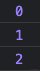```js import { ref, watchEffect } from 'vue';

const a = ref(0);

watchEffect(() => console.log(a.value));

a.value++; a.value++; ```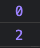```js import { ref, effect } from '@vue/reactivity';

const a = ref(0);

effect(() => console.log(a.value));

a.value++; a.value++; ``````js import { signal, effect, batch } from "@preact/signals-core";

const a = signal(0);

effect(() => console.log(a.value));

batch(() => { a.value++; a.value++; }) ```

`@vue/reactivity`中要想要達到同樣效果的話… 關鍵是這個`@vue/reactivity`連個文件都沒有！`Vue`官網上的`Composition API`是又封裝了一層，用法已經不一樣了。比方說`Composition API`裡的`watch``@vue/reactivity`裡就沒有，而且`watchEffect``effect`表現也不太一致，`@vue/reactivity``README`寫的也特別簡陋：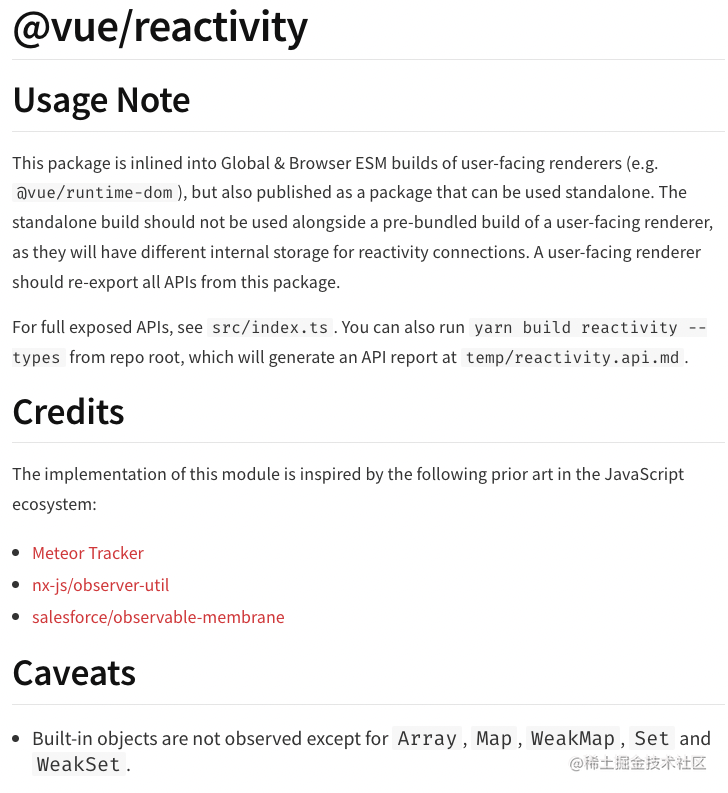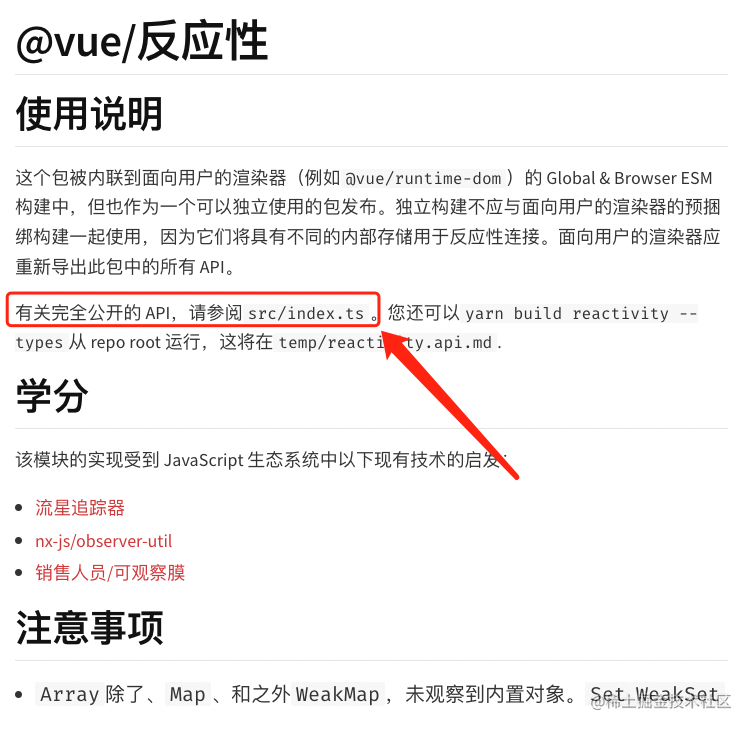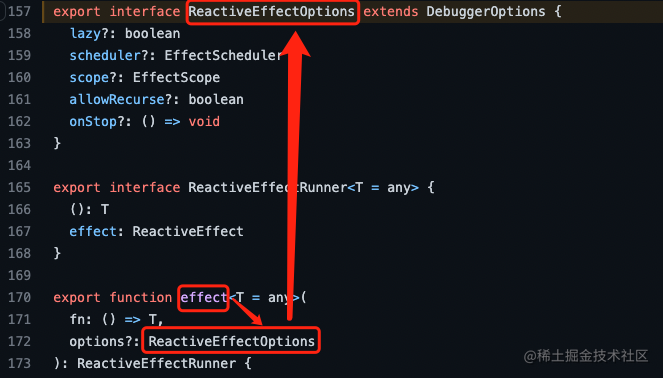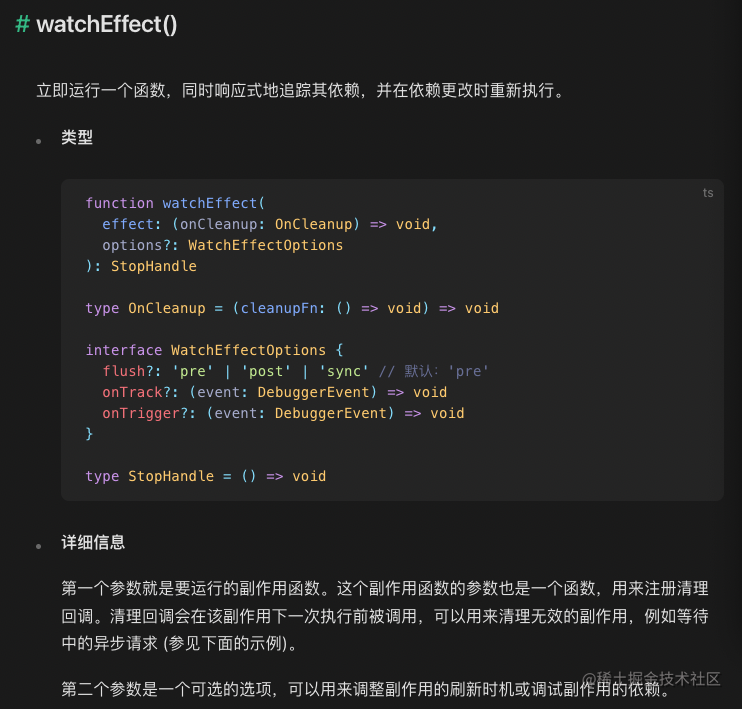```js import { ref, effect } from "@vue/reactivity";

const a = ref(0);

effect(() => console.log(a.value), { lazy: true });

a.value++; a.value++; ```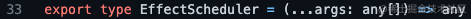```js import { signal, computed, effect, batch } from "@preact/signals-core";

const counter = signal(0); const double = computed(() => counter.value * 2); const tripple = computed(() => counter.value * 3);

effect(() => console.log(double.value, tripple.value));

batch(() => { counter.value = 1; // Logs: 2, despite being inside batch, but `tripple` // will only update once the callback is complete console.log(double.value); }); // Now we reached the end of the batch and call the effect ```

`batch`函式還可以巢狀著寫：

```js import { signal, computed, effect, batch } from "@preact/signals-core";

const counter = signal(0); effect(() => console.log(counter.value));

batch(() => { batch(() => { // Signal is invalidated, but update is not flushed because // we're still inside another batch counter.value = 1; });

// Still not updated... }); // Now the callback completed and we'll trigger the effect. ```

## React 及 Preact

`core`的核心部分講完了，那就繼續看看`@preact/signals`以及`@preact/signals-react`吧！它倆用法都一樣：

```js import { signal } from "@preact/signals-react";

const count = signal(0);

function CounterValue() { // Whenver the `count` signal is updated, we'll // re-render this component automatically for you return

Value: {count.value}

; } ```

```js import { useSignal, useComputed } from "@preact/signals-react";

function Counter() { const count = useSignal(0); const double = useComputed(() => count.value * 2);

return ( ); } ```

# 後續

```js import { ref, effect } from '@vue/reactivity'

const a = ref(0) const fn = effect(() => console.log(a.value), { lazy: true })

a.value++ fn() ```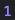```js import { ref, effect } from '@vue/reactivity'

const a = ref(0) effect( () => console.log(a.value), { scheduler (fn) { console.log(fn) } } )

a.value++ ``` 列印結果：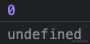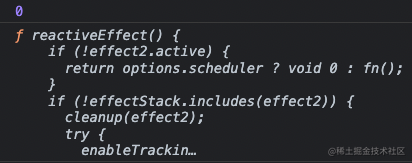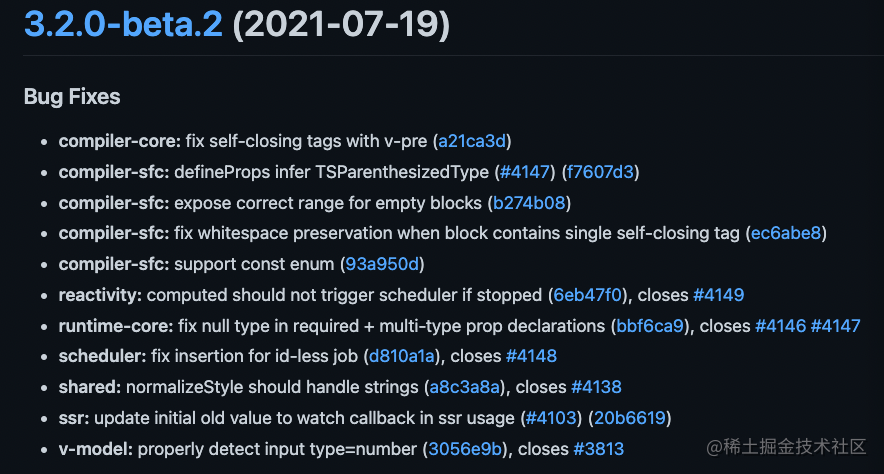```js // 3.2 以前 effect( () => {}, { scheduler (fn) { console.log(fn) } } )

// 3.2 之後（含 3.2） const fn = effect( () => {}, { scheduler () { console.log(fn) } } ) ```

```js import { ref, effect } from '@vue/reactivity'

const jobQueue = new Set() const p = Promise.resolve()

let isFlushing = false function flushJob() { if (isFlushing) return

isFlushing = true

p.then(() => { jobQueue.forEach(job => job()) }).finally(() => { isFlushing = false }) }

const a = ref(0) const fn = effect( () => console.log(a.value), { scheduler () { jobQueue.add(fn) flushJob() } } )

a.value++ a.value++ ``````js import { ref, effect } from '@vue/reactivity'

const a = ref(0) const fn = effect( () => console.log(a.value), { scheduler (func) { console.log(fn === func) } } )

a.value++ ```